# Modeling interfacial electrochemistry: concepts and tools†

Anja Kopač Lautara, Arthur Hagopianbc and Jean-Sébastien Filhol*bc
aDepartment of Materials Chemistry, National Institute of Chemistry, Slovenia
bICGM, University of Montpellier, CNRS, ENSCM, Montpellier, France. E-mail: jean-sebastien.filhol@umontpellier.fr
cRS2E French Network on Electrochemical Energy Storage, FR5439, Amiens, France

Received 11th December 2019 , Accepted 18th February 2020

First published on 21st February 2020

This paper presents a grand canonical formalism to treat electrochemical effects at interfaces. This general formalism is linked with the classical chemical hydrogen electrode (CHE) approximation and an improved approximation is proposed. This new approximation including a higher order correction that (i) keeps the low computational cost of classical CHE approach, (ii) does not require to know the type of reaction (electrochemical/not electrochemical) and (iii) should give better estimates in many problematic cases. Beyond the applicability domain of this new approximation, the homogeneous background method (HBM) which is a potential dependent density functional theory method is then presented. HBM allows computing and extracting, at ab initio level, electrochemical properties of molecules either adsorbed or in the double layer. In particular, quantitative redox potential and number of exchanged electrons can be computed giving access to non-integer electron exchange or decoupled electron/proton transfer reactions. Tools for rationalizing electrochemical reactivity consisting of potential dependant projected density of states, Fukui function and metallicity index are defined. The methodology and tools are applied to examples relevant to the energy domain in order the compare reactivity in the outer Helmholtz plane and at the surface. Then, the combination of HBM and reactivity create a toolbox usable to predict and investigate the different redox, degradation and ageing processes occurring at an electrochemical interface such as the one found in energy materials but also in all electrochemical applications.

## Introduction

Understanding the electrochemical processes occurring at electrode/electrolyte interfaces is critical for many applications ranging from batteries, metal synthesis and purification (e.g. aluminium, zinc…) to corrosion prevention. These processes are often crucial in improving performance, efficiency, safety, and/or cycling of electrochemical devices. However, probing the interface is an experimental challenge, as only few suitable methods exist.1–7 Most of the theoretical methods focus either on bulk electrode or on bulk electrolyte.8–18 As an example, solvation structures,8–10,13–15,17 dynamical properties,10,14,15 ion pair formation,9,13,17 and stability of the system8–11,13,14,16,18 in the bulk electrolyte were studied using ab initio calculations and classical molecular dynamics. These approaches provide a way to better understand bulk electrolyte thermodynamics and dynamics as a function of the electrolyte components and concentration. However, they are usually not applicable to interfacial electrochemistry: at the biased electrode surface, within the inner Helmholtz plane, electric field can reach a value as high as 109 V m−1. This leads to electron exchange between surface and both, adsorbed molecules, and molecules in the double layer region. Electrochemical effects are thus not confined to the electrode surface as they can also influence reactivity (electrolyte, redox species…) in the outer Helmholtz plane (OHP), i.e. over a couple of nanometres from the surface, depending on the salt and solvent concentration.19 In the OHP, electric fields can polarize the electronic structure of molecules and electrons can still be exchanged with the electrode through electron diffusion or tunnelling. All these effects have to be considered as they strongly affect the thermodynamics and kinetics of the different (electro)chemical steps.20,21 The global electrochemical reactivity at electrode is the balance between all these processes that are different in origin, but can still be treated in a unified way.

Herein, we present the general frame of an electrochemical formalism associated with a grand canonical description to account for the surface potential variation: the main energy parameter is the electrochemical free energy. In this framework, we show how redox equilibrium potential and the number of exchanged electrons can be extracted to obtain redox equations relevant to interfaces. We then demonstrate the connection of this general description with a classical approximation, i.e. Nørskov Computational Hydrogen Electrode (CHE) approximation in electrocatalysis.22 This classical CHE approximation considers electrochemical effects in a perturbative way without accounting for the electrode response. We propose an improved approximation including higher order effects at no additional computational cost. We then describe how the free electrochemical energy can be extracted from ab initio calculations, fully taking into account the electric field and potential effects by using the homogeneous background method (HBM) developed previously.23–25 This framework is applied to simple systems relevant to energy applications to illustrate how specific interfacial electrochemical behaviour (e.g. a non-integer number of exchanged electron) can be rationalized and linked to classical Nernst theory. Then, we present general electrochemical descriptors such as the potential dependant Fukui function and projected density of states (PDOS). They provide tools to determine the active redox centre at a given potential and predict its electrochemical behaviour.26–28 Finally, we discuss metallicity, a new electrochemical reactivity index. It allows investigating the competition between chemistry/catalysis and electrochemistry at the interface as it quantifies whether an elementary step is associated with a purely electrochemical reaction, a purely chemical/catalytic one (i.e. independent of the potential), or an intermediate one.

The approaches presented herein provide general methodologies to deal with electrochemical systems within perturbative or ab initio framework. They can be used to investigate the reactivity of molecules either in the inner or outer Helmholtz plane as a function of potential, but also to quantitatively predict redox properties and reaction products.

## Grand canonical approach to interface electrochemistry

In contrast to bulk, the free energy of surfaces and interfaces not only depends on the chemical composition (xi), temperature T, and pressure p, but also on the surface charge Qs and the applied potential V.23,24 Both charge and potential stand for additional non-independent degrees of freedom linked by the surface differential capacitance, that is related to the surface chemical hardness.26,29 The potential change is an intrinsic response property of the surface charge for a given interface. Then, interfacial electrochemistry requires the DFT calculations to be coupled with the Grand canonical approach (vide infra). This allows the electrode/electrolyte interactions to be considered quantitatively. In the Grand canonical ensemble, the relevant energy is the grand potential that is herein called the free electrochemical energy F((xi),T,p,V(Ne)). It links the Gibbs free energy G(xi,T,p,Ne) of the electrochemical system to an electron reservoir set at a potential V as follows:
 F((xi),T,p,V(Ne)) = G((xi),T,p,V(Ne)) + Ne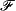V(Ne) (1)
where Ne is the number of excess electrons relatively to surface neutrality andis the Faraday constant. Graphical representation of the free electrochemical energy as a function of potential resembles an inverted parabola. The top of parabola corresponds to the uncharged surface and thus gives the energy of the uncharged system E0 and the associated “potential of zero-charge” V0 (Fig. 1). For potentials lower than V0, the surface is negatively charged, while it is positively charged for higher potentials. Note that the free electrochemical energy of the interface behaves similarly as the interfacial surface tension, and therefore its graph resembles electrocapillarity curves.30 This shape is directly linked to the second order expansion of the surface free electrochemical energy and is given by:26
 (2)
where C is the surface capacitance (at zero charge). The surface charge Qs is related to the potential by: QsC(VV0).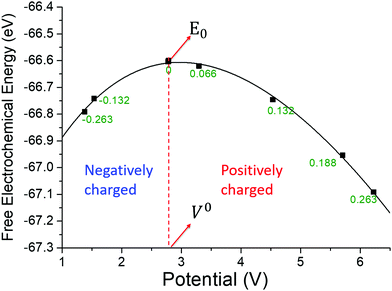Fig. 1 Free electrochemical energy of a water monolayer on Pd(111) surface as a function of potential.31 The dotted line gives the position of the potential of zero charge V0 for this surface and separates the positively charged and negatively charged surface domains. The green numbers are the corresponding charge of the surface at the given potential.

When investigating a surface electrochemical reaction, a redox pair of surfaces has to be considered: one for the reduced form and one for the oxidized (Fig. 2). At each potential, the relative stability of the oxidized and reduced interfaces is given by the one with the lowest free energy. From the graphical representation, the equilibrium potential of the electrochemical reaction (Veq) is directly deduced from the crossing-point of the two parabolas (Fox = Fred) (Fig. 2). The number of electrons displaced along the interfacial reaction is given by: ΔNe = Nred(Veq) − Nox(Veq), where Nred(Veq) and Nox(Veq) are the number of electrons needed for both the reduced Sred and the oxidized Sox interfaces to reach Veq from the uncharged surfaces. Thus, the half reaction associated with the surface Sox/Sred redox pair is:

 Sox + ΔNee− ⇄ Sred (3)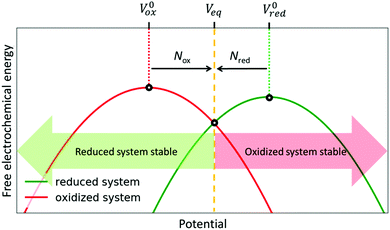Fig. 2 Generic representation of the potential-dependent free electrochemical energy for a redox pair of one reduced system Sred (green) and one oxidized system Sox (red). To reach the equilibrium potential, electrons have to be added (subtracted) to the reduced (oxidized) system, respectively. In general, the number of added and subtracted electrons is not the same (Nox ≠ Nred ≠ 0). The two systems coexist only at equilibrium potential (i.e. redox potential), determined as the crossing of the two F–V curves. At potentials different from equilibrium potential, the thermodynamically stable system is the one with lower free electrochemical energy.

Note that for a redox process at the surface ΔNe does not have to be an integer. This surprising non-Nernstian phenomenon is valid only in the half-cell for a given interfacial elementary step and will be discussed in detail (vide infra).

### CHE approximation and beyond

A popular approximation used in electro-catalysis to obtain potential dependant reaction paths was introduced by Nørskov as the Computational Hydrogen Electrode (CHE) method.22 This method only uses uncharged surface calculations and corrects the electrochemical effect by adding an energy term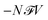to account for the electric work contribution. The correction is associated with the number of exchanged electrons N and the electrode potential V that is referenced to the hydrogen electrode, giving name to the method. However, the method is based on a few strong hypotheses. The first assumption is that the ongoing redox process is well defined and is concomitant with the exchange of a charged redox specie that was H+ in the original paper (e.g.: S + e + H+ ⇄ S–H). The second assumption is that surface reaction steps can be separated into two groups: the reaction is either an electrochemical step associated with an (integer) number of exchanged electrons, or it is a purely catalytic/chemical step, independent of potential. But, from an atomistic perspective, the reaction type can be ambiguous as a purely acidic reaction (e.g.: S + H+ ⇄ S–H) could be considered instead of an electrochemical one. In the given example, the discrimination between these two types formally depends on the charge state of the surface. Unfortunately, as the atomic charge is not an observable in quantum mechanics, some ambiguity always remains.

To investigate the CHE assumptions and limits, we give the connection between CHE approximation and the Grand canonical approach. CHE only considers the energetics of uncharged interfaces: the energy variation ΔFCHE(V) is computed between uncharged initial and final states (Fig. 3). The initial state corresponds to the non-protonated bare interface S with energy E0S, a proton H+ in a reservoir with a chemical potential set to the one of H in H2 (by the hydrogen electrode reference), and an electron in a reservoir with an electrochemical potential μe = −eV, with V the potential of the electrode. The final state is the protonated reduced surface S–H with an energy E0S–H. The energy variation is then:

 (4)
with VVH+/H2 the electrode potential referred to the standard hydrogen electrode.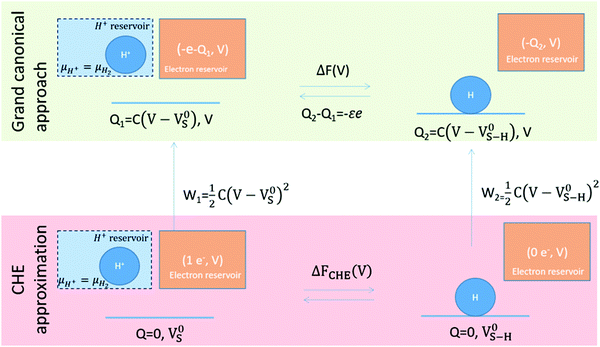Fig. 3 Thermodynamic cycle linking the free electrochemical energy in the grand canonical approach ΔF(V) and in the classical CHE approximation ΔFCHE(V).

Nevertheless, the system considered in the CHE, leading to eqn (4) is strictly non-physical as the potentials of reduced and oxidized interfaces correspond to their zero charge potentials (that are different): thus, they have no reason to correspond to the imposed potential V, in opposite to the thermodynamic equilibrium condition. The physical system consists of charged interfaces (reduced and oxidized) at the same potential V, but with different surface charges Q1 and Q2 (Fig. 2). Therefore, the electron reservoir, the reduced, and the oxidized system are all at a common potential V. The physical system and the CHE system can be linked through the thermodynamic cycle given in Fig. 3. The equation ΔF(V) = ΔFCHE(V) + W2 − W1 can be extracted, with W1 and W2 being the electrical work that is necessary to change the potentials of reduced and oxidized interfaces from their zero-charge potential V0 to the common potential V. Therefore, the CHE approximation is only valid when W2W1 ≈ 0.

A more advanced approximation can be made by using the second order expansion of the free electrochemical energy given in eqn (2):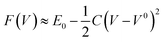. Assuming that the capacitance is similar for both interfaces, the expansion at second order gives eqn (5) (detailed derivation of eqn (5) can be found in ESI (S.0)):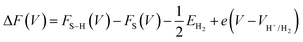(5)
with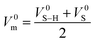and εe = C(V0S–HV0S). We can rewrite eqn (5) into:
 ΔF(V) ≈ ΔFCHE(V) + εe(V − V0m) ≈ ΔFCHE(V) + C(V0S–H − V0S)(V − V0m) (6)

Eqn (5) shows that the need to get S and S–H interface at the same potential V induces a supplementary term εe associated with the different intrinsic potential of zero charge of the two surfaces forming the redox pair. Therefore, the CHE is exact at second order only if ε ≈ 0 corresponding to V0S–HV0S. It has been shown in previous papers that this is not a general case, as the zero charge potential can be shifted by many volts with only slight reorganization of the surface.22,32 An other vision of this same phenomena is given when eqn (6) is rewritten as ΔF(V) ≈ ΔFCHE(V) − Δp0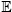with the surface dipole p0 = −SV0ε024,33,34 and the surface average electric field= Qt/0 and C(VV0m) = Qt. Then, the CHE approximation fails when a strong change in the surface dipole occurs with the reaction and modifies the interaction with the local electric field as shown in ref. 35 and 36. This explains why the CHE approximation can fail in the case of a reaction step associated with a strong change in the potential of zero charge of the interface (or equivalently of the surface dipole) while it is reasonable when the surface zero charge potentials remain mostly unchanged upon reduction/oxidation.

A more general correction could easily be obtained from the uncharged calculations, at nearly no additional cost, by first extracting the potential of zero charge (that can be computed similarly to the surface work function). Then, assuming a surface capacitance in the experimental range (e.g., 10–20 μF cm−2 for water electrolytes) the corrective C(V0S–HV0S)(VV0m) term is calculated. Furthermore, the number of exchanged electrons 1 + ε can be obtained from this extended approach as ε = C(V0SV0S–H)/e. For ε ≈ −1, the step can be considered non-electrochemical. Contrarily, |ε| ≈ 0 is associated with an electrochemical process. Thus, this extended CHE approximation can be used to separate chemical and electrochemical steps.

The impact of this new correction can be investigated by comparison with a previous potential dependant study of water reorientation on Pd(111) surface.31 The CHE would consider this reorientation as non-electrochemical and therefore whatever the potential is, ΔFCHE(V) = ΔE0 with ΔE0 ≈ −0.08 eV. The zero-charge potential of the two water orientations gives V0m = 3.9 V vs. vacuum and ΔV0 = 2.1 V. In this case, the correction at a potential of V = 5 V vs. vacuum (0.6 V vs. SHE) is C(V0S–HV0S)(VV0m) ≈ 0.29 eV and ΔF(V) ≈ −0.21 eV The model correctly reproduces the stability inversion of the two water structures and the computed value for the free electrochemical energy is close to the one obtained from the potential dependant ab initio calculation (−0.14 eV). Similarly, the equilibrium potential can be computed to 4.2 V vs. vacuum that is close to the ab initio value (4.5 V vs. vacuum).

This correction extends the applicability of the CHE approximation to many problematic cases; it does not necessitate to guess the nature of the electrochemical process (electrochemical vs. non-electrochemical) and provides realistic results. Nevertheless, if this approximation gives reasonable results, it is not always precise enough as the free electrochemical energy can diverge from the parabolic ideal model. In this case, free electrochemical energy needs to be computed at ab initio level as a function of the applied potential.

### Computing electrochemical interface: the homogeneous background method

Many methods were proposed to calculate the potential dependent free electrochemical energy.37–39 For example, the reaction center can be represented by an atomistic cluster while varying electronic charge and reaction coordinates.40–49 Studying orientation of the water molecules near the interface at different potentials was done by describing the metal by jellium model and developing tight binding molecular dynamics method.50–59 A homogeneous countercharge and a potential reference was used to vary the potential and investigate the processes happening at the interface.23–25,60–64 The homogeneous background method initially proposed by Filhol and Neurock and then refined is computationally affordable, numerically stable and easily applicable in all periodic DFT codes.23,24 It enables retrieving electrochemical descriptors from DFT calculations and it is applicable to a wide potential range. Thus, it is a powerful yet simple method for introducing potential dependence in DFT calculations. Herein we give some details on its practical use.

Within the homogeneous background method, the surface potential is adjusted by explicitly adding or withdrawing electrons from the unit cell of the DFT calculation. Homogeneous distribution of opposite charge over the entire unit cell (i.e., homogeneous background) is added to compensate for the added/withdrawn electrons. This keeps the overall unit cell neutral, as required by the periodic boundary conditions to avoid electrostatic energy from diverging.23–25,60,65–68 The homogeneous background creates an electrostatic potential profile that models the electrochemical double layer in the solvent region. In this way, it simulates the presence of the ionic distribution in the vicinity of the interface.60,65–68 However, some corrections have to be applied in order to account for the introduction of non-physical contributions to the description of the system. These corrections were addressed previously and are presented in detail in the ESI (S1).24,25,28,32 Herein, we only present the general reasoning. The chemical potential of the homogeneous background contributes to the total computed DFT energy EDFT(Ne). The electronic energy Ee is then obtained by:

 (7)
where Va is the potential averaged over the unit cell, and the entire second term in eqn (7) represents the correction of the chemical potential of the homogeneous background. Furthermore, as the homogeneous background is distributed over the entire unit cell, it results in the bulk charging of the metal slab. To account for this, a fraction of the added/withdrawn electrons is used to screen the background charge in the metal slab (Nbulk), and a fraction of the added/withdrawn electrons is used for tuning the potential of the system (Nactive):24
 (8)
where d0 is the vacuum thickness of the unit cell and d is the size of the entire unit-cell. The corrected electronic energy is then:
 (9)
where E0DFT is the DFT energy computed at potential of zero charge, i.e. at Ne = 0.

The electrochemical potential of the interface is determined directly from the DFT calculation, independently from the energy corrections, by evaluating the position of the Fermi level relatively to vacuum position (similar to the classical evaluation of a surface work function). As previously shown,24 a vacuum reference potential can easily be extracted in a symmetric unit cell as it would correspond to an extremum of the Hartree potential in the middle of the vacuum part of the unit cell. When using implicit solvent, this reference remains correct as by symmetry there is no electric field at the middle of the vacuum and therefore no polarization of the continuous medium. Importantly, the consistency of the method and of the different corrections can be checked thought the fundamental thermodynamic relation linking surface energy, surface charge and electrochemical potential (that are independently computed):

 (10)

The corrected electronic energy (eqn (9)) can then be used to compute the electrochemical free energy within the grand canonical approach using (eqn (1)):

 (11)

Overall, the homogeneous background method can be considered as a computationally affordable way of introducing potential into the calculations while giving physically and chemically relevant results. It was successfully used in different systems confirming its applicability to a wide potential range.25,27,29 An example of HBM applicability is given herein for the reduction into Mg0 of Mg2+ solvated by dimethyl ether (DME) at Mg interface. This example is technologically important for multivalent battery technology as Mg battery are investigated as cheaper, safer and high energy density alternative to Li-ion. It also illustrates the specific reactivity of molecules in the outer Helmholtz plane.27 The computed curves are given in Fig. 4. Computational details for all the following HBM calculations can be found in ESI (S2, Fig. S1). In this case the free energy curve representative of Mg2+ is quite anharmonic close to the equilibrium potential and presents a rather different capacitance than the Mg0. The CHE approximation and the proposed extension are therefore not valid, and the free electrochemical energy needs to be explicitly computed as function of potential. This behavior is the consequence of the strong electronic reorganization of Mg2+ solvation sphere with potential, inducing important change of differential capacitance.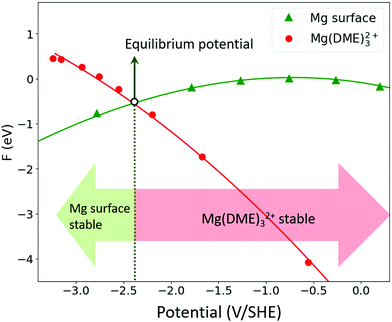Fig. 4 The computed free electrochemical energy for the Mg0 surface (reduced system) and Mg2+ solvated in DME (oxidized surface) as a function of potential.

The calculated reduction potential −2.39 V vs. SHE is remarkably close to the experimental value −2.37 V vs. SHE.69 Note that except the implicit solvent parameters, this is purely a ab initio result without any experimental parameter needed.

### Connection between surface electrochemistry and Nernst limit

For the Mg2+/Mg interface, the global charge at the equilibrium potential for the oxidized interface is +1.6e and −0.6e for the reduced one. Furthermore, for the oxidized interface, the charge on the surface and on the solvated Mg2+ can be directly integrated from the charge density and are found to be −0.4e and +2e, respectively (Fig. S2, ESI). Thus, the electrochemical equation of the redox pair Mg0/Mg2+ can be directly extracted as:
 Mg−0.4surface + Mg(DME)32+ + 2.2e− ⇄ Mg−0.6surface + Mg0 + 3DME, Veq = −2.39 V vs. SHE (12)

The number of exchanged electrons is then 2.2, which does not exactly correspond to the expected 2 electrons from the classical Nernstian approach that considers that the end members of a redox pair have defined integer charge states.

This seems to strictly contradict the non-locality principle of electrons in quantum chemistry for extended systems. Nevertheless, in the dilute reference used in the Nernst approach, Mg2+ can be considered isolated, not interacting with the electrode (no electrostatic or chemical interaction): this allows defining a zone in the solvent large enough to recover the 2+ charge even if the charge is partially delocalized on the solvation shell. In the case of the interface, Mg2+ is interacting with the surface. Consequently, there is some coupling between the Mg redox state and the surface as this interaction is modifying the electrical properties and in particular the surface potential through the image charge. Therefore, some fraction of charge has to be added per unit cell: this charge compensates the potential shift induced by the Mg2+–surface interaction to maintain the oxidized and reduced surfaces at the same equilibrium potential (Fig. 5).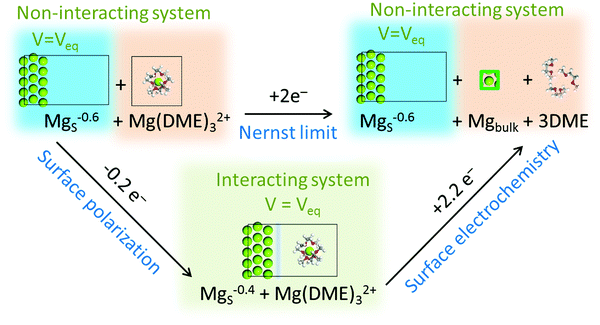Fig. 5 Thermodynamic cycle linking the global Nernstian process to elementary electrochemical interfacial reaction steps.

Thus, in any surface electrochemical step, the number of exchanged electrons does not have to be an integer. Nevertheless, the electrochemical process global balance over all steps should still give the Nernst limit. As an example, the global Mg2+ reduction into Mg0 is associated with two electrons exchanged, but this reduction can be decomposed into two surface steps (Fig. 5). The first step corresponds to Mg2+ reaching the electrode and interacting with it. This interaction is changing the surface potential and 0.2e has to be removed from the surface to maintain it at Veq. In the second step, two electrons have to be provided for the Mg2+ reduction at the surface, but an extra 0.2 electron is also needed to maintain the potential at Veq. The global Nernst balance is then 2 exchanged electrons, but each reaction step at the interface is associated with a non-integer electron exchange because an extra fraction of electron (per unit of surface) has to be added to maintain the interface potential constant. Furthermore, when considering an electrode at a constant potential, all reaction steps, even the non-electrochemical ones, will strictly necessitate some electron exchange and will strictly depend on the potential. This can explain the non-Nernstian shifts that were associated with fractional electron transfer in some surface reaction.70

### Extensions and limits of the homogeneous background method

The HBM was developed for metal/electrolyte interface, but can be applied to any surface even of oxide such as rutile RuO271 or perovskites72 while these surfaces remain metallic. In the HBM, the electron transfer (controlled by the potential) can be decoupled from the hydrogen one in opposite to CHE, therefore decoupled reactions can be investigated.72,73 Furthermore, as the method accounts for the electronic reorganization occurring with the electrochemical reaction, it can also account for non-integer electron transfer at an elementary step and should help to understand non-Nernstian systems.74,75 Nevertheless, as any model, the HBM has some limitations. First, it assumes that the capacitance is dominated by the interface and therefore implies that the electrolyte concentration is high (that is the case in most practical systems). Low concentration electrolyte would necessitate inclusion of a Gouy–Chapman-like approach in the implicit electrolyte description. Then, the HBM as a DFT method also includes DFT limits (strong correlation…) and gives time-independent solutions for electrons to the Schrödinger problem. It is then oblivious of electron kinetic: electronic structure is always in the ground state and the electron reorganization is instantaneous. Thus, HBM hypothesizes that the kinetic of electron transfer is fast and non-limiting for the global electro-kinetics. The electron transfer is indeed usually fast so the approximation should hold most of the time. Nevertheless, if the electrode and the redox center are separated by a thick insulating layer, electron tunneling can become slow and therefore become the limiting step: HBM in its classical version would not be applicable.

## Tools for investigating electrochemical reactivity

Electrochemical reactivity at the interface plays a crucial role, as it can reveal (competitive) reaction pathways of the active redox species, degradation of the electrolyte, and consequently whether the system will work optimally.27 Herein, we discuss three electrochemical descriptors that provide valuable information on the electrochemical reactivity (i.e. the redox center and its evolution), and on the nature of the elementary step (i.e. is it electrochemical, catalytic or intermediate). These three descriptors are potential dependent PDOS, Fukui function, and metallicity index.

### Potential dependent PDOS

Projected density of states is a classical approach to comprehend interface reactivity.76 In electrochemical calculations, PDOS is also a potential dependent descriptor and can be directly extracted. The first example is given for the case of the Mg–Mg(DME)32+ interface. The PDOS on the surface and on the solvated Mg2+ complex can be easily separated. While the shape of each PDOS changes only slightly with potential, their relative position is strongly modified. The PDOS of the surface shows that the relative position of the Mg metal sp-band is shifted with the potential change relatively to the vacuum reference (green in Fig. 6a). Under reduction, electrons added to the surface increase the electron–electron repulsion and destabilize the surface band structure: Fermi level and the entire Mg band shift towards higher energies leading to the surface potential increase (as μe = −eV). In opposite, the PDOS shows that Mg(DME)32+ molecular orbital energies (red in Fig. 6a) remain rather constant relatively to the vacuum reference.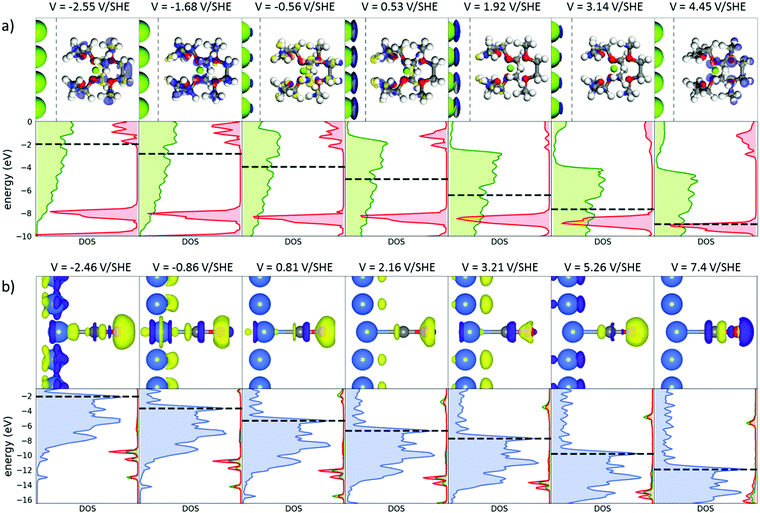Fig. 6 Evolution of Fukui function and the projected density of states as a function of the applied potential for (a) Mg(DME)32+ at a Mg interface and (b) adsorbed CO at the Pt surface. The positive contribution of the Fukui function is given in blue, and the negative in yellow. The PDOS on metal surface is given on the left (green for Mg, blue for Pt), and the PDOS on the molecular specie on the right. All energies are referred to vacuum level. For the sake of visual representation about 5 Å of empty space was removed in the Fukui of Mg(DME)32+ in between the surface and the molecule (dashed line). Note: the potential range used in this study is far larger than the typical stability range of the associated electrolytes but still features how the electronic structure can reorganize with potential.

Another limit case is given by a strongly adsorbed CO molecule on a Pt(111) surface. PDOS gives an evolution of the Pt metal band and Fermi level similar to Mg case. However, in contrast to Mg(DME)32+ the energies of CO molecular orbitals are strongly modified with the change of surface potential (Fig. 6b). This difference is induced by CO molecular orbitals strong mixing with the Pt band: thus CO acquires a partial metallic behaviour.

Similarly, to PDOS, the potential-dependent PDOS can be used to probe the interactions between adsorbate/molecule and the surface. It also provides information on how the molecular orbitals of the adsorbate/molecule are shifted relatively to the metal band with the change of the applied potential. This helps rationalizing the shift in the surface/adsorbate reactivity with potential, as previously shown.31 Nevertheless, potential-dependent PDOS does not provide a direct intuitive insight into the electrochemical reaction. To go beyond this limitation, the Fukui function can be used to determine the redox center and the ongoing electrochemical process using a more chemical approach.

### Potential-dependent Fukui function

Fukui function is a good descriptor for the electrochemical response of a system to a change in the electronic charge/electrode potential.26 It can be extended to a potential dependant form defined as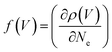, where ρ(V) is the electron density at potential V and Ne the number of electrons (details are given in S3, ESI). Fukui function has a positive (dominant) contribution that can be mainly identified as the redox centre of the electrochemical reaction, i.e. the region of the interface that undergoes charging with the potential change. The negative contribution (associated with a small positive counterpart) corresponds mostly to polarization effects induced by changes in the charge distribution even if it can be sometimes associated to bond reorganization.31 The Fukui function gives an easy visualization of the associated redox centre. Commonly used in molecular quantum chemistry to probe frontier orbitals, it was shown to be a fundamental element of the electrochemical reactivity, as it is directly linked with the potential of zero charge and the capacitance.26 It was also used as a perturbative descriptor of the electrochemical reactivity,24,29 but only computed for the uncharged interface. Herein, it is extended to the grand canonical approach in order to follow the evolution of the redox centre as a function of the potential (Fig. 6). Fukui function is then a suitable tool to predict the regioselectivity of a redox process. As an example, all three DME molecules can be reduced in the Mg(DME)32+ complex, but the Fukui function is mainly localized on the DME molecule on the opposite side to the surface (Fig. 6a and 7). This molecule is therefore expected to be more reactive under reduction than the other two DMEs. This is confirmed at lower potential when this DME undergoes fragmentation into ethylene and methanolate.27,28 The surprise is that the molecule farthest away is the most reactive. This trend seems to be a general behaviour for the molecules in the double layer.27,28 It can be explained by the strong electric field at the interface that is distorting the potential and slightly shifting the molecular orbital energies with the distance to the surface (Fig. 7). In the case of reduction, the farther away a molecule is, the lower its LUMO is, implying that the molecule farthest away is the first to be reduced. However, this effect is moderated by the tunnelling probability of the electron that has an exponential decay with the barrier thickness: if the distance is too large, the kinetics of electron transfer prevents the reduction. Therefore, for thick double layer, when tunnelling kinetics becomes slower than the molecule reorientation/diffusion (i.e. slower than of the order of nanoseconds), the reduced specie would become a mix between the electrochemical response given by Fukui function and tunnelling probability. Nevertheless, for most electrochemical systems where high ionic concentrations are used, the double layer remains quite thin (of the order of one nm) and therefore the tunneling is fast in comparison to the redox center movement. Thus, the electrochemical process should be properly predicted by the Fukui function.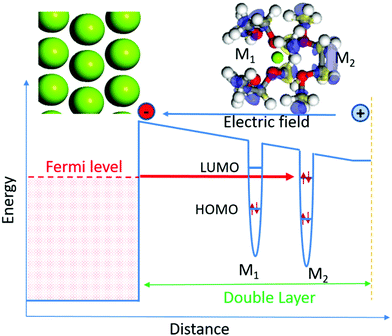Fig. 7 Scheme of the evolution of the potential with the distance to the surface due to the electric field in the double layer and the influence on the HOMO/LUMO energies for the attached DME molecules. Figure shows the case of reduction, where LUMO orbital is lowered with increasing distance from the surface.

### Electrochemical reactivity at the surface or in the double layer: metallicity index

As shown in previous papers, the electrochemical reactivity can occur both at contact point between the surface and the adsorbed species, or in the outer Helmholtz plane.2,27–29,32,49,63,77,78 Examples are presented in Fig. 6 for strongly adsorbed CO on a Pt surface, and for Mg(DME)32+ in the double layer, only weakly interacting with the Mg surface. As seen from the potential dependant PDOS, the addition or subtraction of electrons at the electrochemical interface is associated with a shift of the metal band energy. Importantly, the change in the position of Fermi level relatively to the position of the molecular orbitals and more particularly of highest occupied molecular orbital (HOMO) and lowest unoccupied molecular orbital (LUMO) levels determines the overall interactions and electron transfers: it is the reason for the electrochemical reactivity. Herein, we define the metallicity index as a measure of the change in the position of molecular orbital (Kohn–Sham) levels relatively to Fermi level. A limit case of surface/adsorbate interaction occurs when the electronic structure of the adsorbate and of the surface mix to the point that the adsorbate can be considered as a resonant part of the surface. Then, with applied potential, the adsorbate orbitals will shift like the Fermi level of the surface, i.e. the slopes of the Fermi level vs. potential is equal to −1 by definition (μe = −eV). The corresponding metallicity index would be equal to 1. In the opposite case of a molecule that does not interact with the surface, its orbitals energy position is independent of the applied potential: the metallicity index would be 0. The metallicity index is not exactly a measure of the metallic bond as given by Ayers et al.,79 but a measure of the interaction between the adsorbate and surface.

We define a procedure to obtain such a metallicity index. For each MO of a molecular adsorbate, at each potential, the corresponding MO dominated band energy relatively to vacuum (MO)can be extracted from the PDOS (see Fig. 6). The energy change of the MO position as a function of potential is nearly linear as seen in Fig. 8. The metallicity index for this MO, δ(MO) is: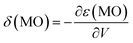. The average metallicity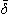for the molecule is then defined as the average value over all the valence orbitals of the molecules.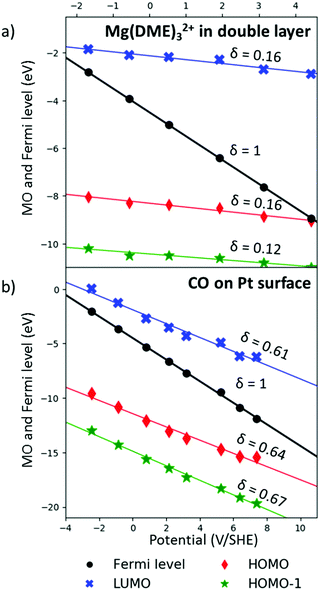Fig. 8 Evolution of the energy position of different molecular orbital levels as a function of the potential (in particular the HOMO, HOMO−1, LUMO and Fermi level) for (a) Mg(DME)32+ in the double layer, and (b) CO adsorbed on the Pt surface. The slope of the lines gives the metallicity index δ. Fermi level has by definition slope of −1, i.e. metallicity of 100%. A metallicity close to zero insures an effective modification of metal/molecule interactions, effective electron transfer from/to the surface Fermi level to/from the active molecule LUMO/HOMO. In opposite, a 100% metallicity implies no electrochemical activity: the MO position is following the surface metal band and therefore the interface–molecule interaction is not modified by the potential. The energies are referred to vacuum level.

In the case of a strongly adsorbed molecule such as CO on Pt surface, the CO MOs are efficiently mixed with the Pt surface d-bands. The position of each of the maxima of the PDOS associated with CO molecular orbitals relatively to vacuum reference were extracted (Fig. 6b) and presented in Fig. 8b. Metallicity for the MOs of CO range from 61% to 67% and the average metallicity is= 64%. This means that when the metal band shifts by −1 eV with a bias of 1 V, the MOs of CO are shifted by −0.64 eV. Thus, the relative interactions governing the chemical bonding between metal band and CO MOs are shifted by 360 meV, only. It is therefore not very efficient to induce a change into CO–surface interactions and reactivity by potential modification. Furthermore, the CO Fukui function for potential below 3.21 V vs. SHE is mostly associated with oxygen non-bonding lone pair (Fig. 6b). Then, the C–O and C–Pt bonds are only weakly modified, meaning that the applied potential is not only inefficient at changing metal–CO interactions (high metallicity), but that the resulting small change does not affect the strong adsorption of CO on Pt surface. This explains the well-known Pt surface poisoning.80–85 Fukui function shows that CO undergoes a change in the active redox centre that shifts from the oxidation of the O lone pair to one of the C–O bond at potential above 5.26 V vs. SHE (Fig. 6b). However, this potential is not reachable for conventional electrochemical experiments because of electrolyte stability limitations.

In contrast to the strongly absorbed CO on Pt surface, we consider Mg(DME)32+ in the double layer, where the distance between the molecule and the surface is large, i.e. approximately 8 Å. In this case, there are no strong chemical interactions between the surface and the redox specie, only long-range electrostatics: the electrochemical process only consists in charge transfer and electric field effect. Therefore, the energies of the different Mg(DME)32+ MOs are only weakly changed with the applied potential (Fig. 6a and 8a), in agreement with the average Mg(DME)32+ metallicity that remains small (= 15%). In this case, the applied potential efficiently modifies the interaction (in particular electron exchange) between the surface and the molecule. This translates into the capability to have a strong modification of the electrochemical properties with a rather small potential change. This was more closely investigated using Fukui and PDOS. When Fermi level is between the highest occupied molecular orbital and the lowest unoccupied molecular orbital of Mg(DME)32+ (corresponding to potential between −1.7 and 3.2 V vs. SHE), the electrons localize mainly on the surface, inducing only a modification of the surface potential (Fig. 6a). The contribution of the Fukui function on Mg(DME)32+ shows small positive (blue) and negative (yellow) contributions linked with the electronic polarization of the molecule induced by the strong local electric field. At reductive potential (V = −2.6 V vs. SHE), Fermi level reaches the LUMO orbital of Mg(DME)32+ and the electrons are transferred from the surface to the Mg(DME)32+. Fukui function determines the redox centre that corresponds to the antibonding σ* molecular orbital of one DME molecule (Fig. 6a and 7). This strongly suggests that reduction will induce a fragmentation of that DME molecule, which has indeed been observed theoretically27,28 and experimentally.86 At oxidative potential (V = 4.5 V vs. SHE), PDOS shows that the Fermi level reaches the HOMO orbital of Mg(DME)32+, and electrons are transferred from Mg(DME)32+ to the surface. The Fukui function demonstrates that electrons are withdrawn from the oxygen lone pair of the DME molecule. This observation is in agreement with the oxygen activation that leads to the formation of peroxide, which is a known problem of glymes under oxidation.87–89

In the outer Helmholtz plane of the double layer, metallicity is low as the active redox MOs are not interacting strongly with the electrode surface orbitals. Therefore, the redox reaction is controlled by the position of Fermi level relatively to the HOMO/LUMO frontier orbitals (whose energy is almost constant), and the associated electron transfer between the electrode and the redox centre. In this case, the reactivity is mostly electrochemically controlled, and does not depend much on the nature of the electrode.

If the reaction is occurring in the inner Helmholtz plane with an adsorbed molecule on the electrode surface, the reactivity is different because of the metallization of the adsorbate. In the case of 100% metallicity, the potential change of the interface has no effect on the interactions between the adsorbate and the surface. The associated reaction step would then be non-electrochemical and would correspond to a purely chemical/catalytic-like effect of the surface. For lower metallicity, the interaction of the surface and the active specie is still modified by the potential and the associated reaction is an electro-catalytic step. Metallicity can therefore be a good criteria to define the nature of an elementary step as electrochemical, electrocatalytic or purely catalytic.

## Conclusion

In this paper, we have shown that interface electrochemical reaction can be modeled and computed by using a grand-canonical approach based on the free electrochemical energy. The connection to the approximate CHE approach is given and an improved version including surface electrochemical response is presented. This approach can be applied at nearly no additional computational cost, using only uncharged classical ab initio calculations, and should extend CHE approximation to usually problematic cases. In the general case, free electrochemical energy can be computed as a function of the potential at DFT level using an approach such as the Homogeneous Background Method. HBM is based on the unit cell charging and a compensating homogenous background countercharge to model the double layer. From the electrochemical formalism and calculations, interfacial redox equations associated with an interface redox pair can be extracted and associated to an equilibrium redox potential. For an interfacial electrochemical step, the amount of electron exchange (per redox specie) is close, but not exactly integer, as extra charge is needed to maintain the surface potential constant upon the electrochemical reaction. Nevertheless, the global electrochemical reaction that is the balance of all elementary steps has to be associated with an integer number of exchanged electron to follow the Nernst limit.

Specific tools can be used to investigate electrochemical reactivity. In particular, the potential dependent PDOS and Fukui function can be used to identify the electroactive center and rationalize its electro-reactivity. Another tool is the concept of metallicity. It allows determining if the considered reaction step is (i) purely electrochemical, associated with electron transfer between electrode and active centre (low metallicity), (ii) purely catalytic with no influence of the potential (high metallicity), or (iii) an intermediate electro-catalytic step.

These approaches and tools allow ab initio computations of quantitative experimental values, such as energetics, redox potential and number of exchanged electrons. The Fukui function rationalizes the electrochemical reactivity by defining redox centres in a quantum mechanics coherent way, while metallicity and potential dependant PDOS predict the type of reactivity. Electrochemical ab initio calculations allow theoretical insight into potential dependent processes happening at the interface, and provide rationalization of experimental data. As such, they present a crucial step in understanding, optimizing or designing complex electrochemical systems, as the ones used for energy storage or conversion, electrodeposition and electrocatalysis.

## Conflicts of interest

There are no conflicts to declare.

## Acknowledgements

JSF and AH thank the French National Research Agency for its financial support through the Labex STORE-EX project (ANR-10LABX-76-01) and the French Research Network on Electrochemical Energy (RS2E). Part of this work was supported by EU through the POROUS4APP project (H2020-NMP-PILOTS-2015 no. 686163). Financial support from the Slovenian Research Agency (research project J2-8167, research core funding P2-0393 and P1-0044) and Honda R&D Europe (Germany) is gratefully acknowledged and appreciated. JSF and AKL also acknowledge the PROTEUS PHP program of Campus France for supporting AKL travelling expenses through the MAGINTER project (No. 40097SG). We gratefully acknowledge Dr Marie-Liesse Doublet and Prof. Dr Robert Dominko for useful discussions. This work was performed using HPC resources from GENCI-CINES (Grant 2019-A0060910369).

## References

1. R. Mohtadi and F. Mizuno, Beilstein J. Nanotechnol., 2014, 5, 1291 CrossRef PubMed.
2. Y. Yu, A. Baskin, C. Valero-Vidal, N. T. Hahn, Q. Liu, K. R. Zavadil, B. W. Eichhorn, D. Prendergast and E. J. Crumlin, Chem. Mater., 2017, 29, 8504–8512 CrossRef CAS.
3. E. M. Erickson, E. Markevich, G. Salitra, D. Sharon, D. Hirshberg, E. de la Llave, I. Shterenberg, A. Rozenman, A. Frimer and D. Aurbach, J. Electrochem. Soc., 2015, 162, 2424–2438 CrossRef.
4. O. Tutusaus, R. Mohtadi, N. Singh, T. S. Arthur and F. Mizuno, ACS Energy Lett., 2016, 2, 224–229 CrossRef.
5. M. R. Nellist, F. A. L. Laskowski, J. Qiu, H. Hajibabaei, K. Sivula, T. W. Hamann and S. W. Boettcher, Nat. Energy, 2018, 3, 46 CrossRef CAS.
6. S. Drvarič Talian, J. Moškon, R. Dominko and M. Gaberšček, ACS Appl. Mater. Interfaces, 2017, 9, 29760–29770 CrossRef PubMed.
7. D. Y. Kim, Y. Lim, B. Roy, Y. G. Ryu and S. S. Lee, Phys. Chem. Chem. Phys., 2014, 16, 25789–25798 RSC.
8. N. Pour, Y. Gofer, D. T. Major and D. Aurbach, J. Am. Chem. Soc., 2011, 133, 6270–6278 CrossRef CAS.
9. S. H. Lapidus, N. N. Rajput, X. Qu, K. W. Chapman, K. A. Persson and P. J. Chupas, Phys. Chem. Chem. Phys., 2014, 16, 21941–21945 RSC.
10. P. Kubisiak and A. Eilmes, J. Phys. Chem. C, 2018, 122, 12615–12622 CrossRef CAS.
11. S. P. Ong, O. Andreussi, Y. Wu, N. Marzari and G. Ceder, Chem. Mater., 2011, 23, 2979–2986 CrossRef CAS.
12. Y. Wang, S. Nakamura, A. Makoto Ue and P. B. Balbuena, J. Am. Chem. Soc., 2001, 123, 11708–11718 CrossRef CAS PubMed.
13. N. N. Rajput, X. Qu, N. Sa, A. K. Burrell and K. A. Persson, J. Am. Chem. Soc., 2015, 137, 3411–3420 CrossRef CAS.
14. J. Z. Hu, N. N. Rajput, C. Wan, Y. Shao, X. Deng, N. R. Jaegers, M. Hu, Y. Chen, Y. Shin, J. Monk, Z. Chen, Z. Qin, K. T. Mueller, J. Liu and K. A. Persson, Nano Energy, 2018, 46, 436–446 CrossRef CAS.
15. N. Sa, N. N. Rajput, H. Wang, B. Key, M. Ferrandon, V. Srinivasan, K. A. Persson, A. K. Burrell and J. T. Vaughey, RSC Adv., 2016, 6, 113663 RSC.
16. E. Jónsson and P. Johansson, Phys. Chem. Chem. Phys., 2015, 17, 3697–3703 RSC.
17. K. M. Callahan, N. N. Casillas-Ituarte, M. Roeselova, H. C. Allen and D. J. Tobias, J. Phys. Chem. A, 2010, 114, 5141–5148 CrossRef CAS.
18. S. Dong, Y. Kim, S.-S. Lee, D. Y. Kim, Y. Lim, B. Roy and Y.-G. Ryu, Phys. Chem. Chem. Phys., 2014, 16, 25789–25798 RSC.
19. W. Schmickler and E. Santos, Interfacial Electrochemistry, Springer, 2nd edn, 2010 Search PubMed.
20. A. J. Bard, H. D. Abruña, C. E. Chidsey, L. R. Faulkner, S. W. Feldberg, K. Itaya, M. Majda, O. Melroy, R. W. Murray, M. D. Porter, M. P. Soriaga and H. S. White, J. Phys. Chem., 1993, 97, 7147–7173 CrossRef CAS.
21. J. Bard and L. R. Faulkner, Electrochemical Methods: Fundamentals and Applications, Wiley, Weinheim 2000, ISBN: 978-0-471-04372-0 Search PubMed.
22. J. K. Nørskov, J. Rossmeisl, A. Logadottir, L. Lindqvist, D. Lyngby and H. Jo, J. Phys. Chem. B, 2004, 108, 17886–17892 CrossRef.
23. J.-S. Filhol and M. Neurock, Angew. Chem., Int. Ed., 2006, 45, 402–406 CrossRef CAS PubMed.
24. M. Mamatkulov and J. S. Filhol, Phys. Chem. Chem. Phys., 2011, 13, 7675–7684 RSC.
25. C. D. Taylor, S. A. Wasileski, J. S. Filhol and M. Neurock, Phys. Rev. B: Condens. Matter Mater. Phys., 2006, 73, 165402 CrossRef.
26. J. S. Filhol and M. L. Doublet, J. Phys. Chem. C, 2014, 118, 19023–19031 CrossRef CAS.
27. A. Kopač Lautar, J. Bitenc, T. Rejec, R. Dominko, J.-S. Filhol and M.-L. Doublet, J. Am. Chem. Soc., 2020 DOI:10.1021/jacs.9b12474.
28. A. Kopač Lautar, PhD thesis, University of Ljubljana, 2019.
29. N. Lespes and J.-S. Filhol, J. Chem. Theory Comput., 2015, 11, 3375–3382 CrossRef CAS PubMed.
30. D. C. Grahame, Chem. Rev., 1947, 41, 441–501 CrossRef CAS.
31. J. Filhol and M. Doublet, Catal. Today, 2013, 202, 87–97 CrossRef CAS.
32. J. S. Filhol and M. L. Bocquet, Chem. Phys. Lett., 2007, 438, 203–207 CrossRef CAS.
33. T. C. Leung, C. L. Kao, W. S. Su, Y. J. Feng and C. T. Chan, Phys. Rev. B: Condens. Matter Mater. Phys., 2003, 68, 195408 CrossRef.
34. E. Wigner and J. Bardeen, Phys. Rev., 1935, 48, 84–87 CrossRef CAS.
35. L. D. Chen, M. Urushihara and K. Chan, ACS Catal., 2016, 6, 7133–7139 CrossRef CAS.
36. S. Ringe, K. Chan, S. Ringe and B. Seger, Energy Environ. Sci., 2019, 12, 3001–3014 RSC.
37. J. VandeVondele, R. Ayala, M. Sulpizi and M. Sprik, J. Electroanal. Chem., 2007, 607, 113–120 CrossRef CAS.
38. M. Otani and O. Sugino, Phys. Rev. B: Condens. Matter Mater. Phys., 2006, 73, 1–11 CrossRef.
39. N. Bonnet, I. Dabo and N. Marzari, Electrochim. Acta, 2014, 121, 210–214 CrossRef CAS.
40. A. B. Anderson, Electrochim. Acta, 2003, 48, 3743–3749 CrossRef CAS.
41. T. V. Albu and A. B. Anderson, Electrochim. Acta, 2001, 46, 3001–3013 CrossRef CAS.
42. A. B. Anderson and T. V. Albu, Electrochem. Commun., 1999, 1, 203–206 CrossRef CAS.
43. A. B. Anderson and T. V. Albu, J. Am. Chem. Soc., 1999, 121, 11855–11863 CrossRef CAS.
44. A. B. Anderson and N. C. Debnath, J. Am. Chem. Soc., 1983, 105, 18–22 CrossRef CAS.
45. A. B. Anderson and D. B. Kang, J. Phys. Chem. A, 1998, 102, 5993–5996 CrossRef CAS.
46. A. B. Anderson, N. M. Neshev, R. A. Sidik and P. Shiller, Electrochim. Acta, 2002, 47, 2999–3008 CrossRef CAS.
47. A. B. Anderson and N. K. Ray, J. Phys. Chem., 1982, 86, 488–494 CrossRef CAS.
48. J. Narayanasamy and A. B. Anderson, J. Phys. Chem., 2003, 107, 6898–6901 CrossRef CAS.
49. S. Seong and A. B. Anderson, J. Phys. Chem., 1996, 100, 11744–11747 CrossRef CAS.
50. J. W. Halley and D. Price, Phys. Rev. B: Condens. Matter Mater. Phys., 1987, 35, 9095–9102 CrossRef CAS.
51. J. W. Halley, B. Johnson, D. Price and M. Schwalm, Phys. Rev. B: Condens. Matter Mater. Phys., 1985, 31, 7695–7709 CrossRef CAS PubMed.
52. D. L. Price and J. W. Halley, Phys. Rev. B: Condens. Matter Mater. Phys., 1988, 38, 9357–9367 CrossRef PubMed.
53. W. Schmickler, J. Electroanal. Chem. Interfacial Electrochem., 1983, 150, 19–24 CrossRef CAS.
54. W. Schmickler and D. Henderson, J. Chem. Phys., 1984, 80, 3381–3386 CrossRef CAS.
55. J. W. Halley, B. B. Smith, S. Walbran, L. A. Curtiss, R. O. Rigney, A. Sutjianto, N. C. Hung, R. M. Yonco and Z. Nagy, J. Chem. Phys., 1999, 110, 6538–6552 CrossRef CAS.
56. D. L. Price and J. W. Halley, J. Chem. Phys., 1995, 102, 6603–6612 CrossRef CAS.
57. J. W. Halley, A. Mazzolo, Y. Zhou and D. Price, J. Electroanal. Chem., 1998, 450, 273–280 CrossRef CAS.
58. E. Spohr, Electrochim. Acta, 2003, 49, 23–27 CrossRef CAS.
59. E. Spohr, Solid State Ionics, 2002, 150, 1–12 CrossRef CAS.
60. A. Y. Lozovoi, A. Alavi, J. Kohanoff and R. M. Lynden-Bell, J. Chem. Phys., 2001, 115, 1661–1669 CrossRef CAS.
61. C. G. Sánchez, A. Y. Lozovoi and A. Alavi, Mol. Phys., 2004, 102, 1045–1055 CrossRef.
62. P. J. Feibelman, Phys. Rev. B: Condens. Matter Mater. Phys., 2001, 64, 125403 CrossRef.
63. D. Cao, G.-Q. Lu, A. Wieckowski, S. A. Wasileski and M. Neurock, J. Phys. Chem., 2005, 109, 11622–11633 CrossRef CAS PubMed.
64. T. Dufils, G. Jeanmairet, B. Rotenberg, M. Sprik and M. Salanne, Phys. Rev. Lett., 2019, 123, 195501 CrossRef CAS PubMed.
65. G. Makov and M. C. Payne, Phys. Rev. B: Condens. Matter Mater. Phys., 1995, 51, 4014–4022 CrossRef CAS PubMed.
66. J. M. Rosamilia, J. A. Abys and B. Miller, Electrochim. Acta, 1991, 36, 1203–1208 CrossRef CAS.
67. P. A. Schultz, Phys. Rev. Lett., 2000, 84, 1942–1945 CrossRef CAS PubMed.
68. P. A. Schultz, Phys. Rev. B: Condens. Matter Mater. Phys., 1999, 60, 1551–1554 CrossRef CAS.
69. Standard Reduction Potentials, http://www5.csudh.edu/oliver/chemdata/data-e.htm, accessed 13 June 2019.
70. R. L. Doyle, I. J. Godwin, M. P. Brandon and M. E. G. Lyons, Phys. Chem. Chem. Phys., 2013, 15, 13737–13783 RSC.
71. M. E. G. Lyons and S. Floquet, Phys. Chem. Chem. Phys., 2011, 13, 5314–5335 RSC.
72. W. T. Hong, K. A. Stoerzinger, Y. Lee, L. Giordano, A. Grimaud, A. M. Johnson, J. Hwang and E. J. Crumlin, Energy Environ. Sci., 2017, 10, 2190–2200 RSC.
73. M. T. M. Koper, Top. Catal., 2015, 58, 1153–1158 CrossRef CAS.
74. K. A. Stoerzinger, R. Rao, X. R. Wang, W. T. Hong, M. Christopher, Y. Shao-horn, K. A. Stoerzinger, R. R. Rao, X. R. Wang and W. T. Hong, Chem, 2017, 2, 668–675 CAS.
75. K. Schwarz, B. Xu, Y. Yan and R. Sundararaman, Phys. Chem. Chem. Phys., 2016, 18, 16216–16223 RSC.
76. J. S. Filhol, D. Simon and P. Sautet, J. Am. Chem. Soc., 2004, 126, 3228–3233 CrossRef CAS.
77. Y. Ando, Y. Kawamura, T. Ikeshoji and M. Otani, Chem. Phys. Lett., 2014, 612, 240–244 CrossRef CAS.
78. J. S. Lowe and D. J. Siegel, J. Phys. Chem. C, 2018, 122, 10714–10724 CrossRef CAS.
79. P. W. Ayers and S. Jenkins, Comput. Theor. Chem., 2015, 1053, 112–122 CrossRef CAS.
80. H. Ren, M. P. Humbert, C. A. Menning, J. G. Chen, Y. Shu, U. G. Singh and W.-C. Cheng, Appl. Catal., A, 2010, 375, 303–309 CrossRef CAS.
81. J. Liu, F. R. Lucci, M. Yang, S. Lee, M. D. Marcinkowski, A. J. Therrien, C. T. Williams, E. C. H. Sykes and M. Flytzani-Stephanopoulos, J. Am. Chem. Soc., 2016, 138, 6396–6399 CrossRef CAS PubMed.
82. H. P. Dhar, L. G. Christner, A. K. Kush and H. C. Maru, J. Electrochem. Soc., 1986, 133, 1574 CrossRef CAS.
83. W. Vogel, L. Lundquist, P. Ross and P. Stonehart, Electrochim. Acta, 1975, 20, 79–93 CrossRef CAS.
84. M. Montano, K. Bratlie, M. Salmeron and G. A. Somorjai, J. Am. Chem. Soc., 2006, 128, 13229–13234 CrossRef CAS PubMed.
85. J. Grunes, J. Zhu, M. Yang and G. A. Somorjai, Catal. Lett., 2003, 86, 157–161 CrossRef CAS.
86. X. Chen, T. Hou, B. Li, C. Yan, L. Zhu and C. Guan, Energy Storage Mater., 2017, 8, 194–201 CrossRef.
87. T. Tamura and M. Nakamura, Chem. Lett., 2010, 39, 753–755 CrossRef CAS.
88. S. Tobishima, H. Morimoto, M. Aoki, Y. Saito, T. Inose, T. Fukumoto and T. Kuryu, Electrochim. Acta, 2004, 49, 979–987 CrossRef CAS.
89. K. Yoshida, M. Nakamura, Y. Kazue, N. Tachikawa, S. Tsuzuki, S. Seki, K. Dokko and M. Watanabe, J. Am. Chem. Soc., 2011, 133, 13121–13129 CrossRef CAS PubMed.

### Footnote

Electronic supplementary information (ESI) available. See DOI: 10.1039/c9cp06684e
 This journal is © the Owner Societies 2020